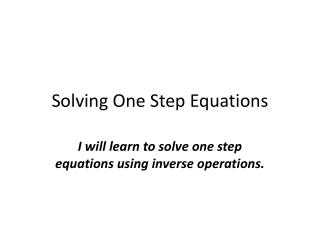# Solving One Step Equations - PowerPoint PPT PresentationDownload PresentationSolving One Step Equations

Solving One Step EquationsDownload Presentation## Solving One Step Equations

- - - - - - - - - - - - - - - - - - - - - - - - - - - E N D - - - - - - - - - - - - - - - - - - - - - - - - - - -
##### Presentation Transcript

1. Solving One Step Equations I will learn to solve one step equations using inverse operations.

2. Steps • Draw a line straight down through the equal sign • Circle the operation being done along with the number • Do the opposite of that operation on both sides of the line • The left side cancels and the variable drops down. Solve what’s on the right and that is your answer. • Check your work by plugging your x value back in!

3. Step 2: Circle the Operation Being Done Along With the Number x + 10 = 20

4. Step 3: Do the Opposite of That Operation on Both Sides of the Line x + 10 = 20 - 10 -10 *Remember that whatever operation you perform on one side of the equal sign, you must do on the other!

5. Step 4: The Left Side Cancels. Bring Down the Variable x + 10 = 20 - 10 -10 X = 10 X = 10 c

6. Step 6: Check Work by Plugging in the Value for X x + 10 = 20 (10) +10 = 20 20 = 20

7. Try It! 1. x – 7 = 5 • 2. x + 8 = 15 3. x + 9 = 30 • Remember, whatever operation you perform on one side of the equal sign you must do to the other! • Also, remember to check your work!

8. Review the Steps! • Draw a line straight down through the equal sign • Circle the operation being done along with the number • Do the opposite of that operation on both sides of the line • The left side cancels and the variable drops down. Solve what’s on the right and that is your answer. • Check your work by plugging your x value back in!